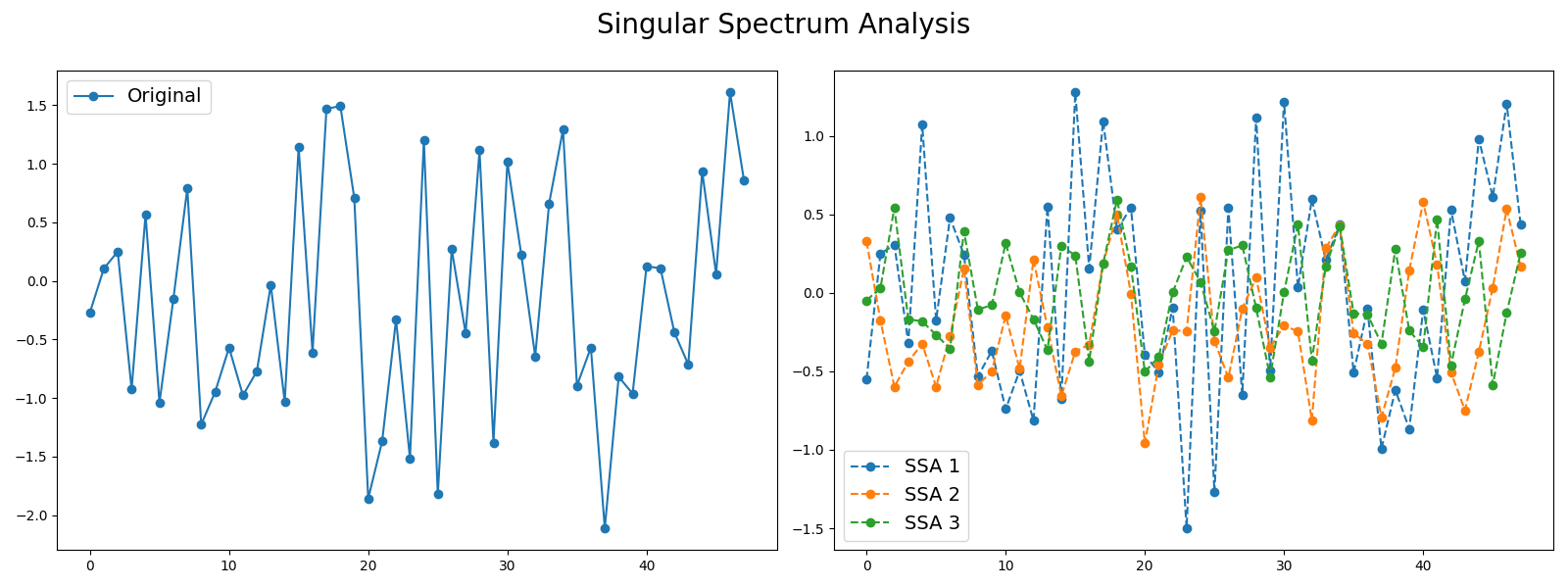# Singular Spectrum Analysis¶

Signals such as time series can be seen as a sum of different signals such as trends and noise. Decomposing time series into several time series can be useful in order to keep the most important information. One decomposition algorithm is Singular Spectrum Analysis. This example illustrates the decomposition of a time series into several subseries using this algorithm and visualizes the different subseries extracted. It is implemented as `pyts.decomposition.SingularSpectrumAnalysis`.```# Author: Johann Faouzi <johann.faouzi@gmail.com>

import numpy as np
import matplotlib.pyplot as plt
from pyts.decomposition import SingularSpectrumAnalysis

# Parameters
n_samples, n_timestamps = 100, 48

# Toy dataset
rng = np.random.RandomState(41)
X = rng.randn(n_samples, n_timestamps)

# We decompose the time series into three subseries
window_size = 15
groups = [np.arange(i, i + 5) for i in range(0, 11, 5)]

# Singular Spectrum Analysis
ssa = SingularSpectrumAnalysis(window_size=15, groups=groups)
X_ssa = ssa.fit_transform(X)

# Show the results for the first time series and its subseries
plt.figure(figsize=(16, 6))

ax1 = plt.subplot(121)
ax1.plot(X, 'o-', label='Original')
ax1.legend(loc='best', fontsize=14)

ax2 = plt.subplot(122)
for i in range(len(groups)):
ax2.plot(X_ssa[0, i], 'o--', label='SSA {0}'.format(i + 1))
ax2.legend(loc='best', fontsize=14)

plt.suptitle('Singular Spectrum Analysis', fontsize=20)

plt.tight_layout()